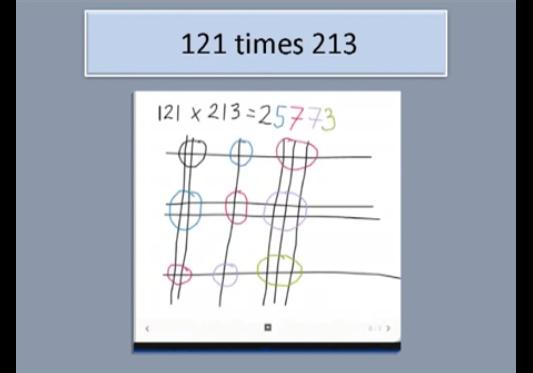# InfoCoBuild

## Computing Mathematics

A series of lectures on computing mathematics, delivered by Tony Mann: Arithmetic by Computer and by Human; How Computers Get It Wrong: 2 + 2 = 5; Proof by Computer and Proof by Human; User Error: Why It's not Your Fault; Finding Stable Matches: The Mathematics of Computer Dating; Might As Well Toss a Coin: How Random Numbers Help Us Find Exact Solutions; This Lecture will Surprise You: When Logic is Illogical; When Math doesn't Work: What We Learn from the Prisoner's Dilemma; and Two Losses Make a Win: How a Physicists Surprised Mathematicians.

Professor Tony Mann has taught mathematics and computing at the University of Greenwich for over twenty years. He was President of the British Society for the History of Mathematics from 2008 to 2011 and is editor of the Newsletter of the London Mathematical Society. (from gresham.ac.uk)Arithmetic by Computer and by Human
Long multiplication, long division and logarithms are, for many, dim-remembered memories, and few now use these skills. We will examine some of the tools that help us, from the abacus to calculators.

How Computers Get It Wrong: 2 + 2 = 5
When lives depend on calculations, human error can kill. From the early days of computing, one problem has been that they generally work with a fixed number of digits, creating rounding errors.

Proof by Computer and Proof by Human
The idea of a proof as a simple, easily-checked method of establishing truth has undergone modification in the age of computers. But the specialisation of the mathematical world has resulted in difficulties with even entirely human-made proofs.

User Error: Why It's not Your Fault
We all make mistakes when we use computer systems - forgetting to save the last document version, neglecting to add the promised email attachment, texting the wrong person, or buying the wrong train ticket.

Finding Stable Matches: The Mathematics of Computer Dating
Computer systems use mathematics to fly aircraft, carry out complex financial transactions and calculate the optimal design for racing cars.

Might As Well Toss a Coin: How Random Numbers Help Us Find Exact Solutions
This lecture looks at the role of randomness in computer algorithm, conducting a live experiment to use random numbers to calculate the mathematical constant π.

This Lecture will Surprise You: When Logic is Illogical
Mathematics offers certainty. That there are infinitely many prime numbers or that four colours suffice to colour any map so that adjacent regions are differently coloured are statements which have been rigorously proved so that there can be no doubt about their truth.

When Math doesn't Work: What We Learn from the Prisoner's Dilemma
Game Theory is a branch of mathematics which tells us how to outthink an opponent or competitor. The Prisoner's Dilemma is a paradox in which knowledge of Game Theory is dangerous: the sophisticated mathematician comes off disastrously worse than the ignoramus.

Two Losses Make a Win: How a Physicists Surprised Mathematicians
One of the most extraordinary pieces of new mathematics was a discovery by the Spanish physicist Juan Parrondo, who was building a computer simulation of an effect in quantum theory.

 Related Links The Story of Maths This is a BBC documentary series written and presented by Professor Marcus du Sautoy, outlining aspects of the history of mathematics. Great Mathematicians, Great Mathematics This is a series of lectures to look at the personality and some of the work of a number of famous mathematicians and then discuss more recent developments and applications. Mathematics for Computer Science This course covers elementary discrete mathematics for computer science and engineering. It emphasizes mathematical definitions and proofs as well as applicable methods.